# Separable process

(diff) ← Older revision | Latest revision (diff) | Newer revision → (diff)

A stochastic process such that the behaviour of its trajectories is essentially determined by their behaviour on a countable subset. A real stochastic process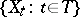defined on a complete probability space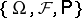, whereis a subset of the real line, is separable relative to a classof subsets ofif there are a countable set(the separant) and a set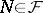,, such that for any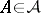and for any open interval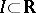one hasHerestands for the intersection.

The concepts of separability relative to the class of closed sets and relative to the class of closed intervals are most important (in the latter case the process is simply called separable). If a processis separable, then for anyand any open set,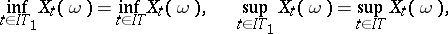(1)(2)(3)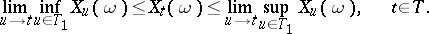(4)

Each of the properties (1)–(4) is equivalent to separability. Ifis a left limit point of, then there is a sequence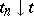of points ofsuch that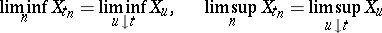with probability 1 (similarly for right limit points). If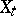is a separable stochastic process that is continuous in probability, then every countable everywhere-dense set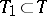is a separant; in addition, for any open interval,, and any sequenceof finite subsets of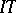satisfying the condition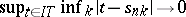, one has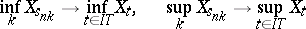(5)

in probability. The convergence in (5) can be replaced by convergence with probability 1 ifis continuous with probability 1.

For any stochastic process,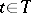, there is a process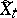,, on the same probability space that is separable relative to the class of closed sets, takes values in the extended real line and is such that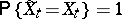,. The concept of separability and its properties can be generalized to processes for whichand the range of values are different general topological spaces. The transition to a separable process enables one to assert the measurability of a number of important functionals and sets connected with a process. An alternative approach consists in extending the-algebra on which it is defined (for example, in the case of a product of Hausdorff compacta, a measure can be uniquely extended from the usual-algebra generated by cylindrical sets to the very rich-algebra of Borel sets), rather than in replacing the random variables constituting the process.

How to Cite This Entry:
Separable process. Encyclopedia of Mathematics. URL: http://encyclopediaofmath.org/index.php?title=Separable_process&oldid=18302
This article was adapted from an original article by V.V. Sazonov (originator), which appeared in Encyclopedia of Mathematics - ISBN 1402006098. See original article